#### Code

##### Downsampling images with filters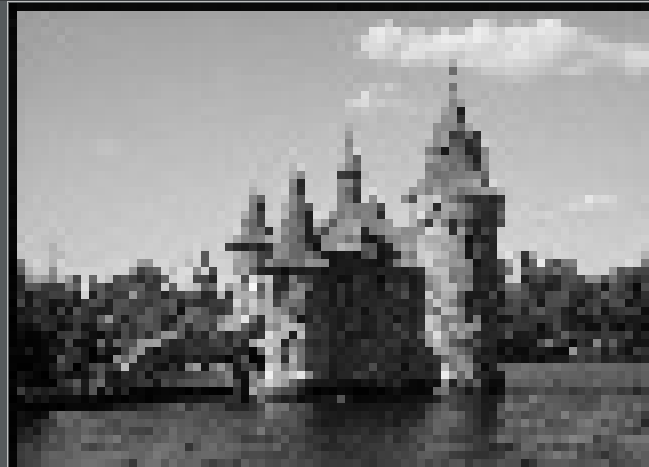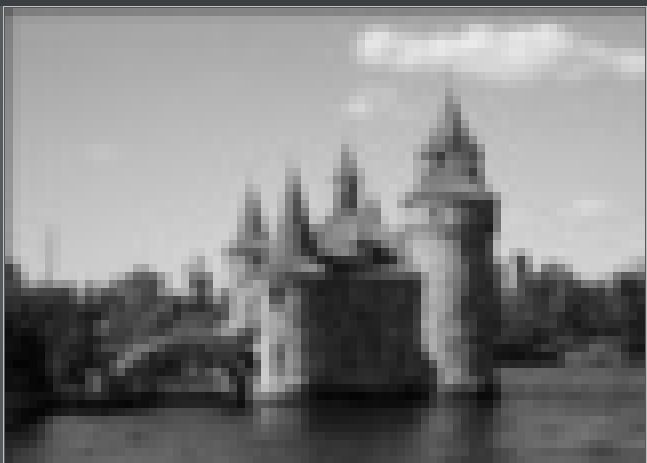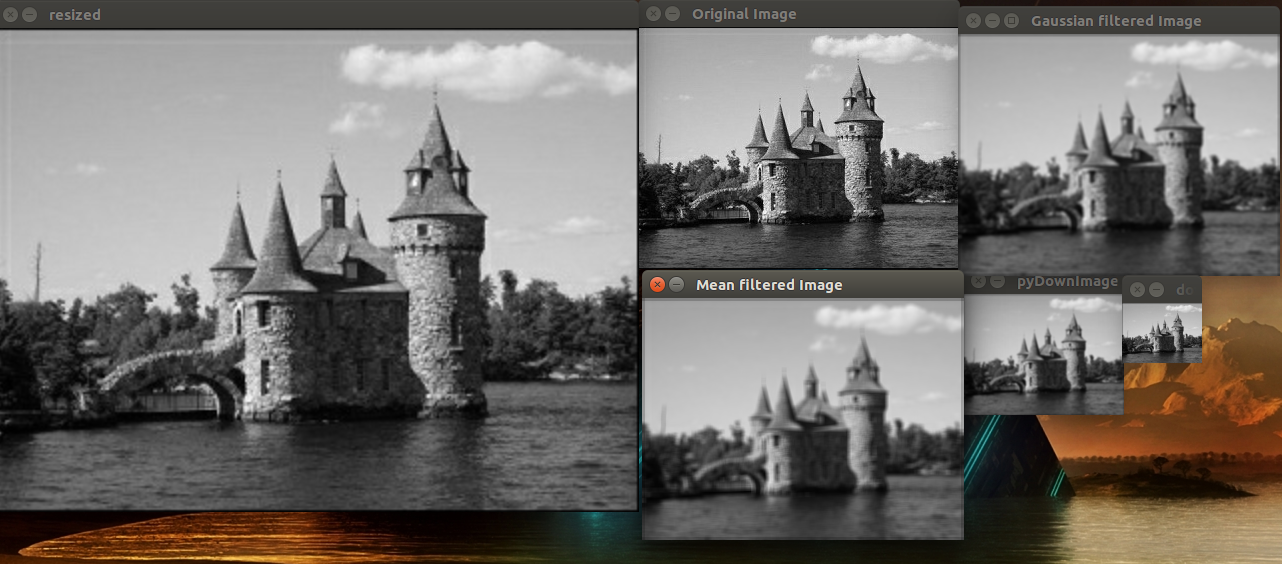median filter（注意其为no-linear）输出：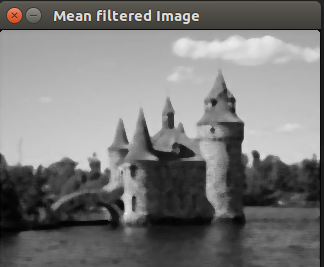##### Applying directional filters to detect edges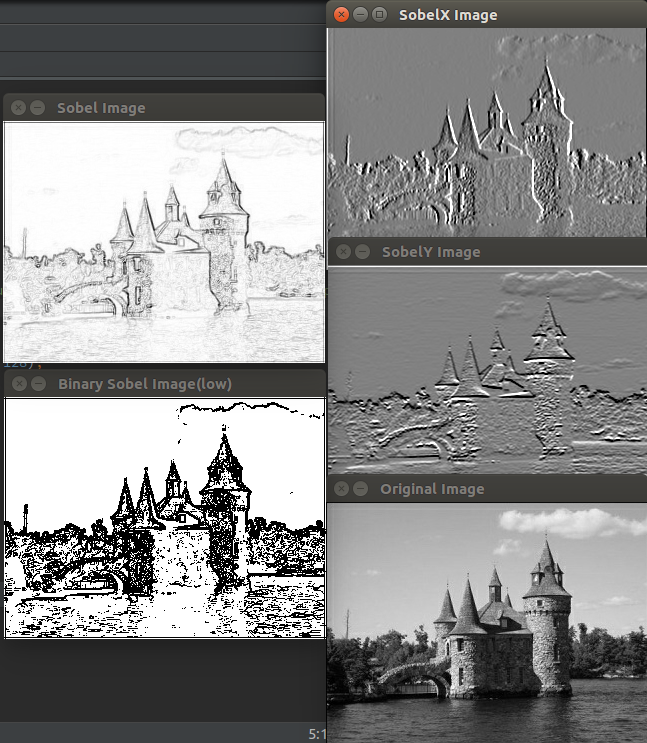##### band-pass filter

cv::GaussianBlur(image, image, cv::Size(5,5), 1.5);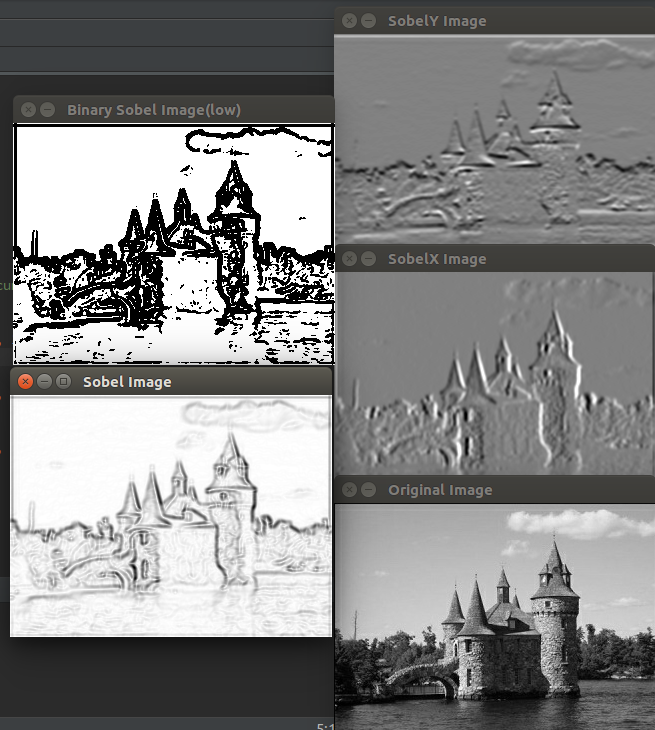##### Computing the Laplacian of an image

ps:这个公式推导不是很懂，所以暂时只是会用…

The Laplacian is another high-pass linear filter that is based on the computation of the image derivatives. As it will be explained, it computes second-order derivatives to measure the curvature of the image function.

Since these larger kernels are computed using the second derivatives of the Gaussian function, the corresponding operator is often called Laplacian of Gaussian (LoG)

. Now, if we subtract the two images that result from the filtering of an image by two Gaussian filters of different bandwidths, then the resulting image will be composed of those higher frequencies that one filter has preserved, and not the other. This operation is called Difference of Gaussians (DoG)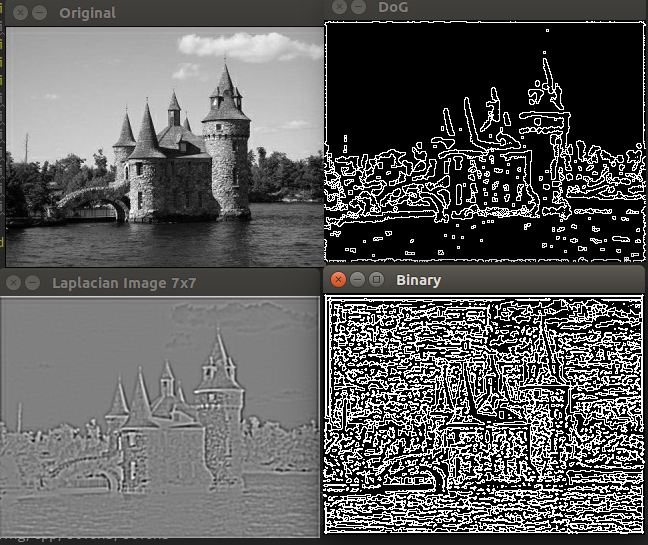《数字图像处理》高教出版社